# R S Aggarwal Solutions for Class 11 Maths Chapter 27 Limits Exercise 27C

Exercise 27C of Chapter 27 is about the limit of a function, which states that, limit of a function exists only when left-hand limits and right-hand limits are equal. Subject experts have prepared all the solutions by keeping listed concepts in mind. Students are advised to download R S Aggarwal Class 11 Solutions and learn more about Limits in detail with the help of quick shortcuts and meticulous presentation of the solutions and check if the function’s limit exists or not. Students can download chapter 27 exercise 27C PDF from RS Aggarwal Solutions from the link given below.

## Download PDF of R S Aggarwal Solutions for Class 11 Maths Chapter 27 Limits Exercise 27C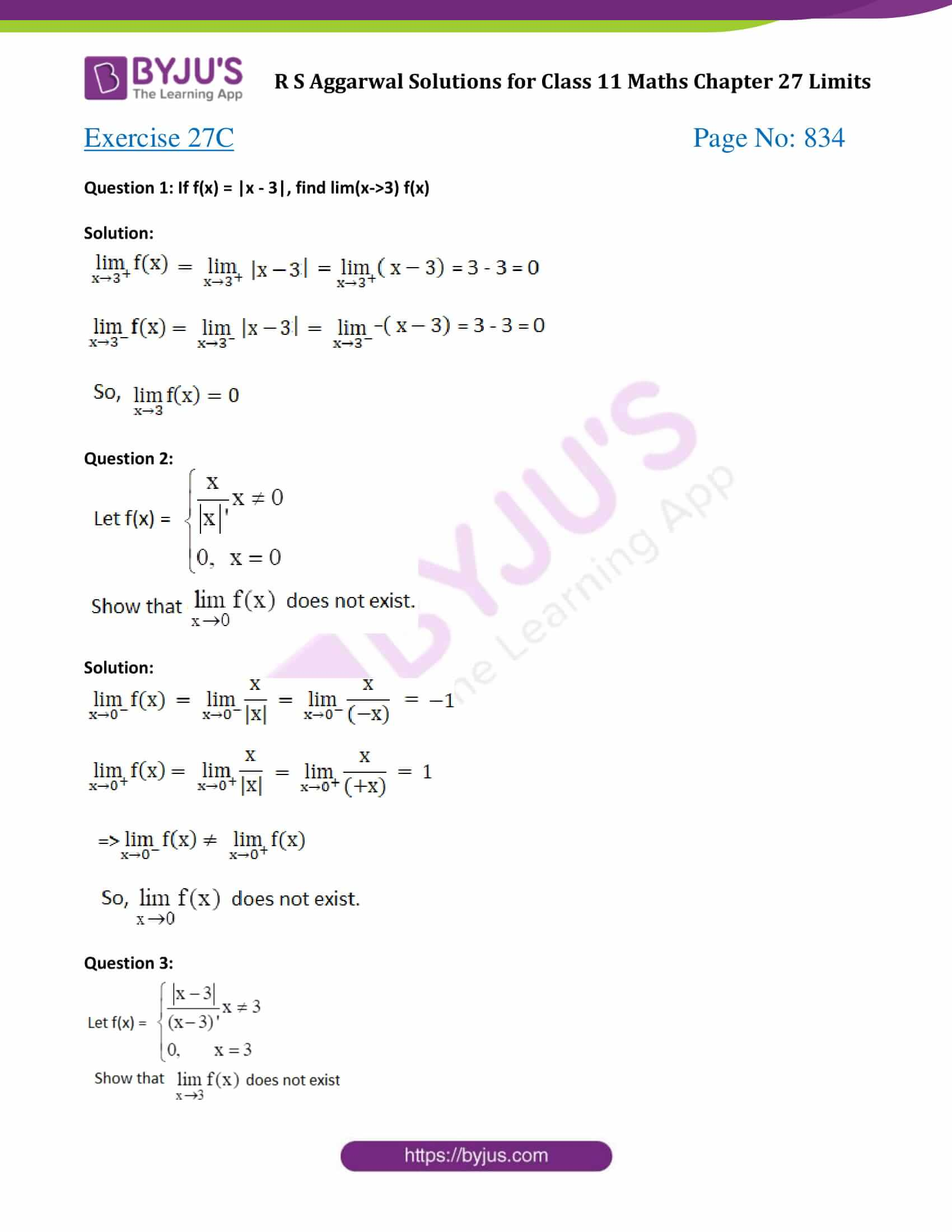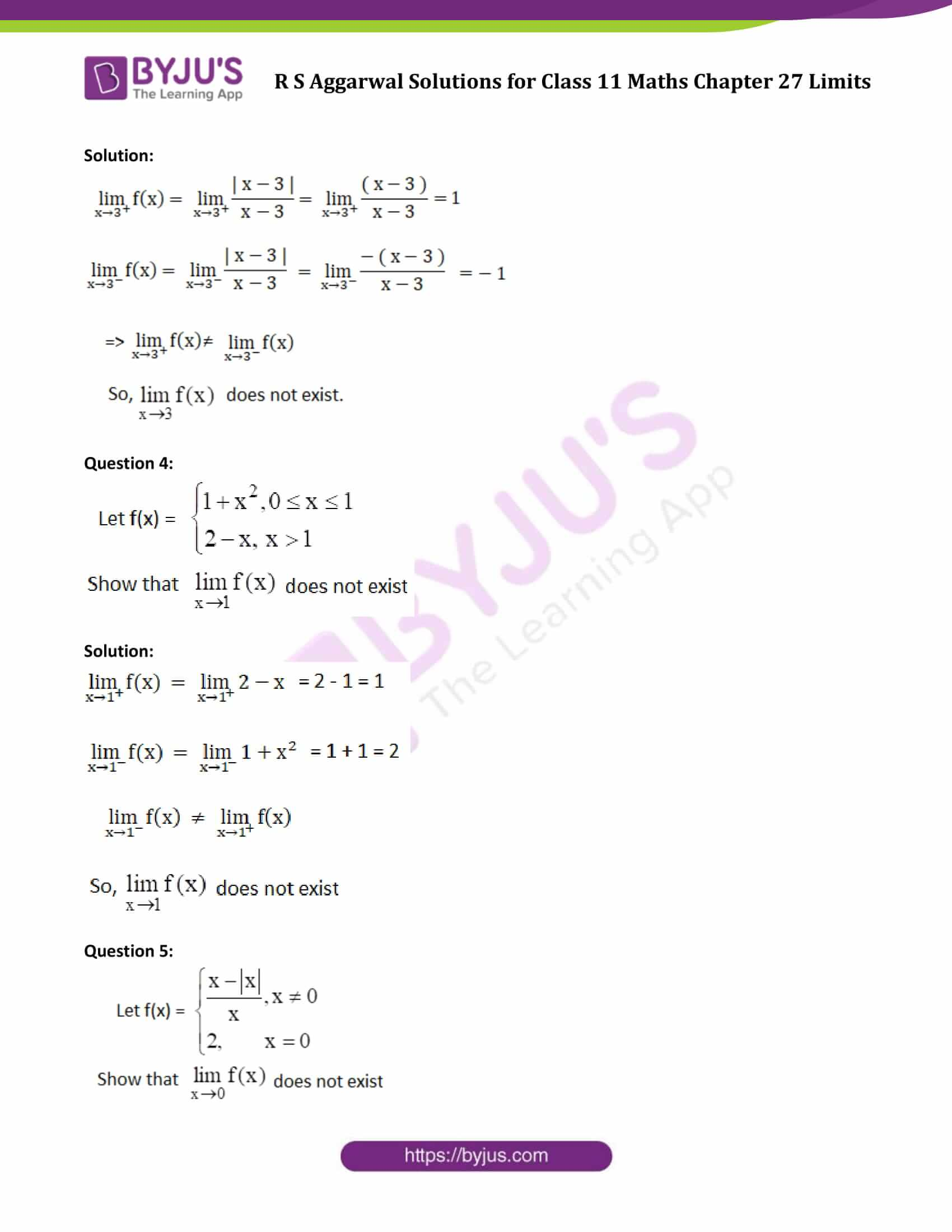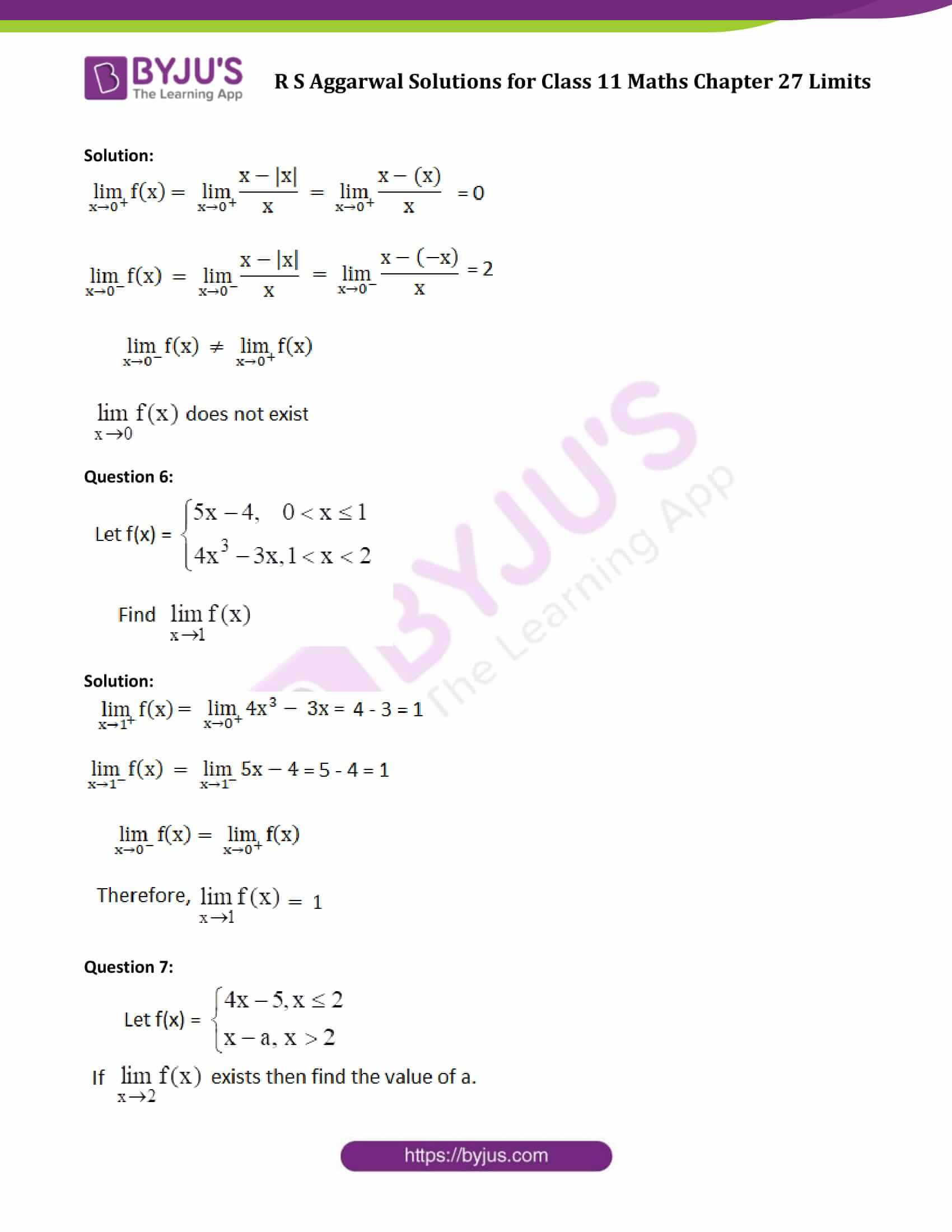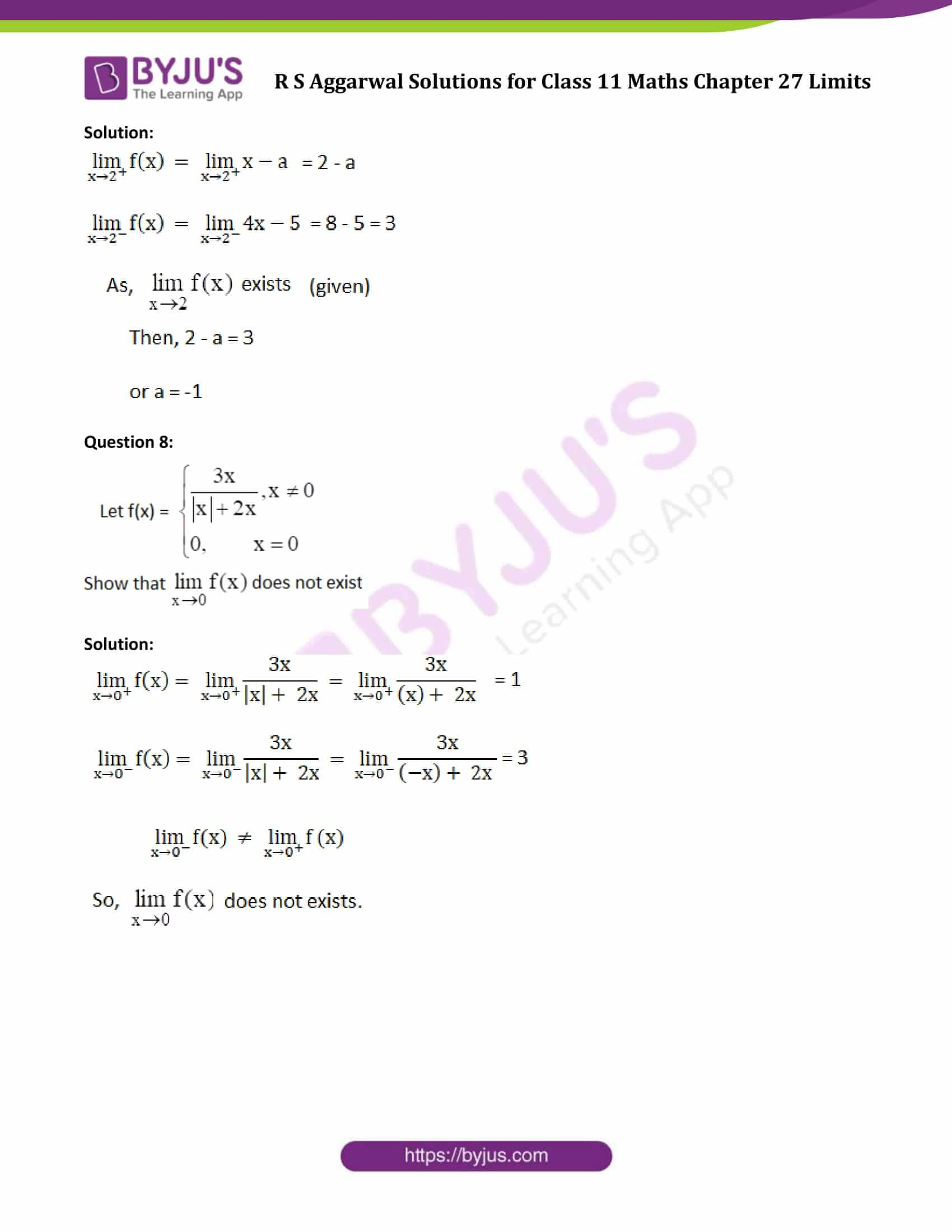### Access Answers of R S Aggarwal Solutions for Class 11 Maths Chapter 27 Limits Exercise 27C Page number 834

Question 1: If f(x) = |x – 3|, find lim(x->3) f(x)

Solution: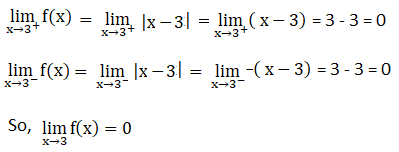Question 2: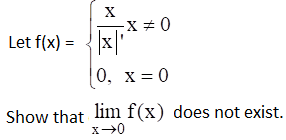Solution: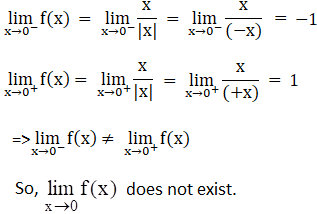Question 3: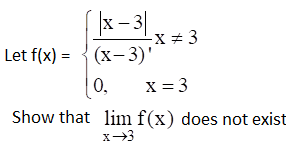Solution: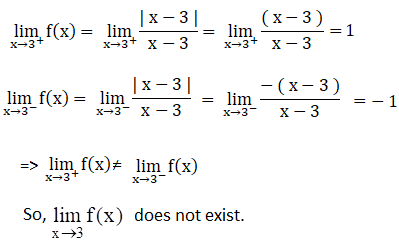Question 4: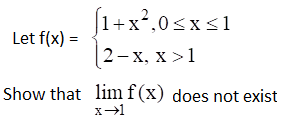Solution: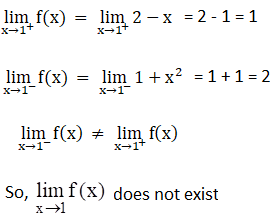Question 5: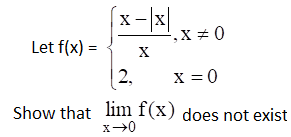Solution: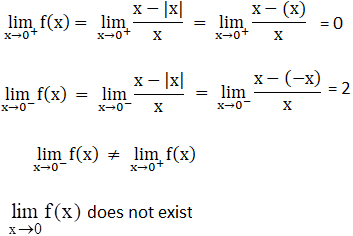Question 6: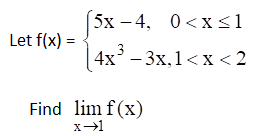Solution: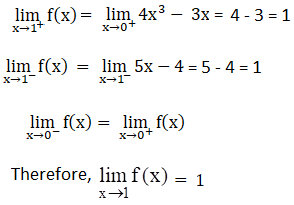Question 7: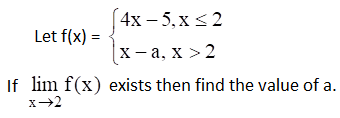Solution: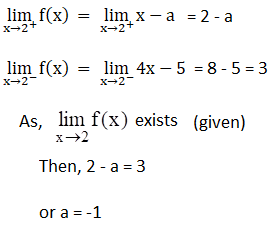Question 8: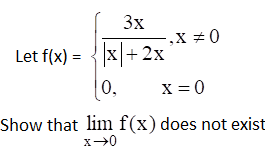Solution: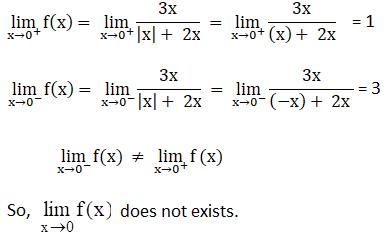## Access other exercise solutions of Class 11 Maths Chapter 27 Limits

Exercise 27A Solutions

Exercise 27B Solutions

## R S Aggarwal Solutions for Class 11 Maths Chapter 27 Exercise 27C

Class 11 Maths Chapter 27 Limits Exercise 27C is based on the following topics:

• Limit of a function
• Left-hand limit
• Right-hand limit